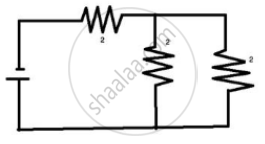# Three Resistors Each of 2ω Are Connected Together So that Their Total Resistance is 3ω. Draw a Diagram to Show this Arrangement and Check It by Calculation. - Physics

Short Note

Three resistors each of 2Ω are connected together so that their total resistance is 3Ω. Draw a diagram to show this arrangement and check it by calculation.

#### Solution 1

A parallel combination of two resistors, in series with one resistor.

R1 = 2 ohm
R2 = 2 ohm
R3 = 2 ohm

1/"R" =1/"R"_1+1/"R"_2

I/"R"=1/2+1/2=1

"R" = "R"+ "R"_3 = 1 + 2 = 3 ohm#### Solution 2

The given diagram of the arrangement is shown in figure in which two resistors are in parallel and then this combination is in series with one resistor.

The equivalent resistance of parallel combination:

R' = (2 xx 2)/(2 + 2) = 4/4 = 1 Omega

Then total resistance of series combinations of R’ = 1Ω with 2Ω resistors is R = 1 + 2 = 3Ω.

Concept: System of Resistors - Resistances in Parallel
Is there an error in this question or solution?

#### APPEARS IN

Selina Concise Physics Class 10 ICSE
Chapter 8 Current Electricity
Exercise 8 (B) | Q 9 | Page 201
ICSE Class 10 Physics
Chapter 7 Electricity
Figure Based Short Answers | Q 9